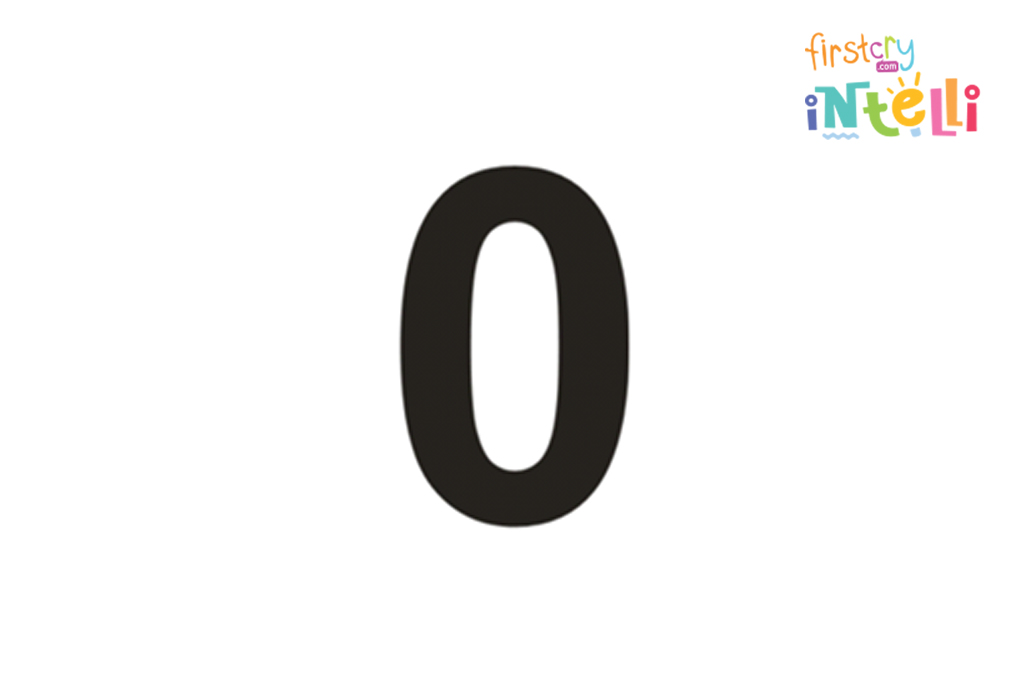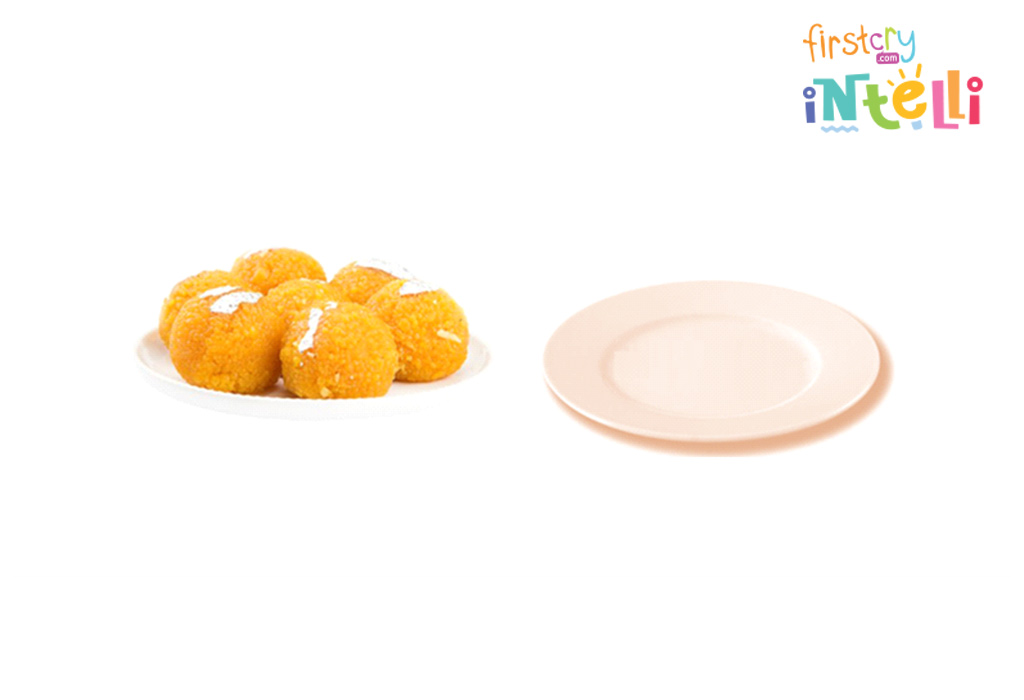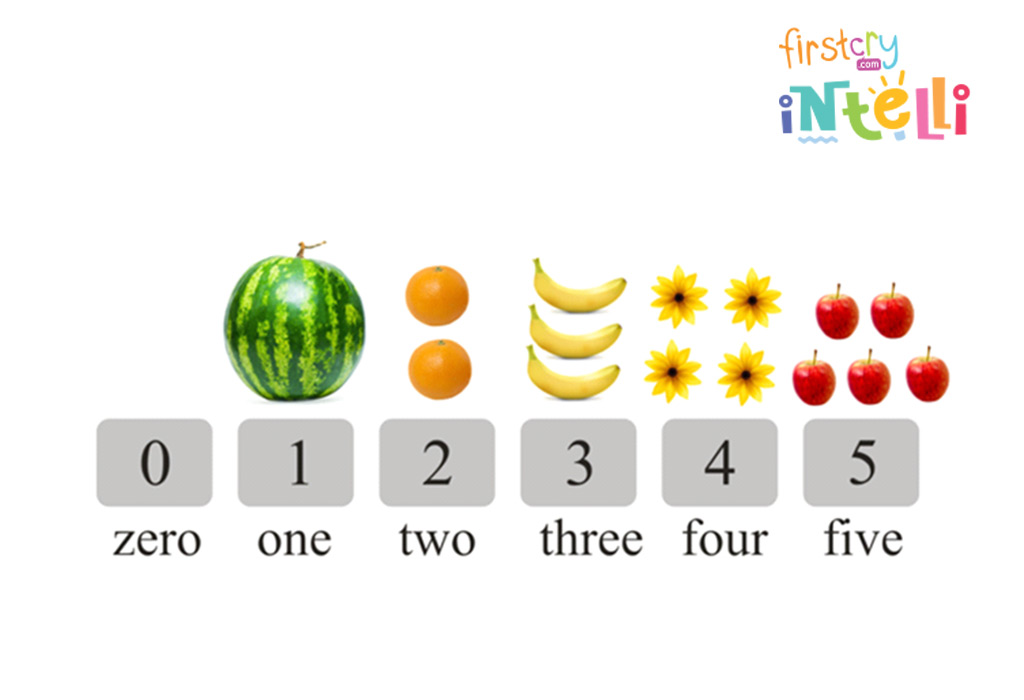HomeBig KidLearning & EducationTeaching Concept Of Zero To Preschoolers And Kids - Importance And Activities

# Teaching Concept Of Zero To Preschoolers And Kids – Importance And Activities

The number 0 might mean nothing, nil, or null, but zero is a very important number in maths. Therefore, it is important that kids must be aware of the concept of zero. You can start teaching your child about this from a young age. So, if you are confused about how to teach the concept of zero to your child, look no further. We have an answer for you in this article.

## What Is Zero?

Zero or 0 is a number with the value null in mathematics. It’s a number that is neither positive nor negative. In simple words, it means empty or nothing. Even though 0 is nothing, it is the most important element in all mathematical calculations. If it is placed before any number, it has no value, but if it is placed after any number, 0 has a significant impact on its value.

## What Is The History Of Number Zero And Who Invented It?

0 is a very special number, and the first recorded zero appeared in Mesopotamia around /(3/) B.C. The concept of zero was introduced by The Mayans, who independently devised it around /(4/) A.D. Zero was then introduced to India in the middle of the 5th Century, reached Cambodia near the end of the 7th Century, and arrived in China and the Islamic countries at the end of the 8th Century. It wasn’t until Arab merchants imported the number in the 11th Century that its use became widespread in Europe. By then, mathematicians in other various parts of the world had already been using it for hundreds of years.

## What Are The Properties Of Zero?

Let’s take a look at the properties of the number zero below.

• Zero is considered an even number.
• The number zero is neither positive nor negative.
• Zero is considered a whole number.
• Zero is considered an integer.
• When we add zero to any number, the result remains the same. Therefore, zero is considered the additive identity for all types of numbers.

## Why Is Learning The Concept Of Zero Important For Children?

Learning the zero concept is important in mathematics as zero is used as a placeholder in place values. For example, there is a huge difference between the numbers 480 and 408, as zero is in different places for both numbers. Therefore, learning the concept of zero is important for children to understand.

## How To Teach Your Child About The Number Zero With Examples

In this section, we will take a look at how to teach kids 0 concept with the help of examples.

For addition with zero, all children need to remember is that when we add zero to any number, the result is the number itself. To understand this, let’s take a look at the examples given below.

• 5 + 0 = 5
• 75+ 0 = 75
• 550 + 0 = 550
• 5265 + 0 = 5265
• 65148 = 0 = 65148

### Subtraction With Zero

For subtraction with zero, all children need to remember is that when we subtract zero from any number, the result is the number itself. To understand this, let’s take a look at the examples given below.

• 7 – 0 = 7
• 85 – 0 = 85
• 647 – 0 = 647
• 9672 – 0 = 9672
• 42679 – 0 = 42679

### Multiplication With Zero

For multiplication with zero, all children need to remember is that when we multiply any number with zero, the result or product is always zero. To understand this, let’s take a look at the examples given below.

• 4 x 0 = 0
• 77 x 0 = 0
• 367 x 0 = 0

### Dividing With Zero

For dividing with zero, all children need to remember is that when we divide zero from any number, the result is always zero. To understand this, let’s take a look at the examples given below.

• 0/6 = 0
• 0/86 = 0
• 0/754 = 754

## How To Representation The Number Zero In The Number Line?

In this section, we will look at the concept of zero in Montessori on a number line.

###On the number line, we put positive numbers on the right of zero and negative numbers on the left of zero. Here zero is an unbiased number, which is neither positive nor negative.

## Number Zero Activities For Preschoolers and Kids

Time to understand the concept of zero with the help of activities for children.

### 1. Play Doug Number

Ask your child to make the shape of 0 using their play dough.

### 2. Trace & Write

On a sheet, mark some dots for your child to trace the zeroes, then ask them to try to write it on their own.

### 3. Find The 0’s

On a drawing sheet, write a lot of numbers, including 0. You can repeat the numbers as well. Ask your child to circle all the 0’s they can spot.

### 4. Mark The 0 Numbers

Give your child a section of the newspaper to read. Ask them to mark all the numbers that have 0 in them. Also, tell them how to differentiate between 0 and O.

### 5. Draw With 0

Ask your child to draw something using the number 0. For example, they can draw a moon, a sun, a snowman, and more.

### 6. Colour The Shape

Make a big 0 on a sheet with a double outline. Ask your little one to fill in the colours within the outline.

Simply flatten the cookie dough and cut it into the shape of 0, using the cookie cutter. With an adult’s help, bake it and start munching!

### 8. 0 Shape Craft

Cut out a huge 0 shape. Give your child some pom poms and guide your child to decorate the shape using colourful pom poms.

### 9. Stamp It Out

Ask your child to collect a few objects that resemble the 0 shapes, like the ends of a foil paper roll, a glass, a pen cap, etc. Help your child dip them in paint and stamp it on paper to make a 0 shape.

### 10. 0 Necklace

Take a few buttons and a thread. Ask your child to add the buttons to the thread and secure it by tying a knot when they are done. Show it to your child and tell them it’s in the shape of 0.

## Simple Tips For Teaching Zero To Your Child

If you think that concept of zero for kindergarten children is a little difficult to understand, we have some simple tips for you to teach your child about this.

• Teach zero to your child after they understand the value of other numbers.
• Make comparisons between zero and nothing, followed by comparing zero with other numbers.
• Explain the concept to them with the help of the activities mentioned above and make the learning process easy and fun for children.

## Interesting Facts About Zero For Kids

• As a placeholder, zero helps to tell the difference between numbers that would otherwise look the same.
• Zero was used for the first time in English in 1598, and it comes from the Italian zero, whose roots are in the Arabic word ṣifr, which means empty.
• Some other names for zero are nil, nought, naught, aught, cipher, and zilch.
• Zero is an even number.
• Zero is a whole number.
• Zero is an integer.
• Zero is a rational number.
• Zero is a real number.

## FAQs

### 1. How To Use of Zero in the Formation of Numbers?

Zero is a number that represents an empty quantity. It also serves as a placeholder for writing numbers, and it extends the theory of numbers from natural numbers to integers.

### 2. Who Found Zero Number in India?

Aryabhatta, a well-known astronomer and mathematician of his time, bought zero in India in the 5th century. Aryabhatta used zero as a placeholder number and introduced zero in the decimal number system and hence, introduced it in mathematics in India.

### 3. Is Zero Odd Or Even Number?

Zero is an even number. When 0 is divided by 2, the resulting quotient is 0—an integer, thereby classifying it as an even number.

### 4. What Type of Number is Zero?

Zero is a whole number.

Learning how to write zero in words and understanding the concept of zero is important for children. We hope this article helped you to teach this concept to your little one.

RELATED ARTICLES